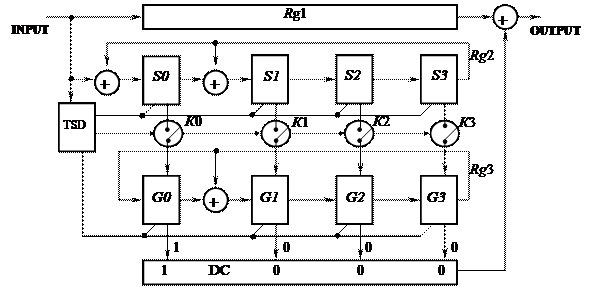CATEGORIES:

# Meggitt decoder of cyclic codes

Meggitt decoder is most acceptable in practice in terms of substantially less complication and providing the best interference protection in comparison to a syndrome decoder.

In the Meggitt decoder, the construction of which is shown in the fig. 1.4, is used register Rg1 for memorizing of the accepted code combination and syndrome register Rg2 with the feedback for the calculation of syndrome {S0, S1, S2, S3}. An additional device is a register Rg3, which is an electronic mechanism for the position-fix of error symbol.Figure 1.4 – Flow diagram of the Meggitt decoder for (15, 11) code

By the signal of the time synchronization device (TSD) after 15 clock cycles, using the keys К0, К1, К2, К3 the content of Rg2 rewrites by the parallel method in Rg3. Detection and correction of errors are produced by the method of linear transformation of syndrome in Rg3 {G0, G1, G2, G3} at l = 14 - j shifts of Rg3, where j is a number of error in the codeword. The value l is determined by the content of Rg2 = {1, 0, 0, 0}. The Rg3 states take values {1, 0, 0, 0} only at the single errors, when the error bit in Rg1 is in output, that is determined by the decipherer (DC).

The construction of Meggitt decoder is simple and is based on the next property of cyclic (n, k) code. If the syndrome

S(x) = F(x) mod P(x),

where F(x) – the code combination registered by a demodulator, corresponds to the error vector

E(x) = xj,

then the content of syndrome register Rg2 S(x), moved on l positions in Rg3 at the condition l = n - j, will be equal S(x) = 1, or, in binary presentation, 1000.

Functional transformations of content of registers Rg1, Rg2 and Rg3 is given below, allow to get a clear idea of principle of the Meggitt decoder work.

Example 1

States of the memory cells of Rg1 of the accepted code combination at the single error:

Rg1 ® 0 0 0 0 1 0 0 0 0 0 0 0 0 0 0,

n = 14, j = 4, l = 14 – j = 10.

 Clock cycle l Rg1 Rg2 Rg3 S0 S1 S2 S3 G0 G1 G2 G3 Ý Correction Ý signal ÝSyndromeÝ

So, on the clock cycle l = 10 the output of Rg3 is 1 0 0 0, in this case the correction signal from DC enters the adder modulo 2, and the single error will be corrected.

Example 2

States of the memory cells of Rg1 of the accepted code combination at the double error:

Rg1 ® 0 0 0 0 1 0 0 0 0 1 0 0 0 0 0,

n = 14, j = 9 (1st error), l = 14 – j = 5.

 Clock cycle l Rg1 Rg2 Rg3 S0 S1 S2 S3 G0 G1 G2 G3 ÝNo correctionÝ signal ÝSyndromeÝ

So, on the clock cycle l = 5 the output of Rg3 is 0 1 1 0, in this case there is no correction signal from DC, and the errors won’t be corrected. However, extra errors in the codeword won’t appear.

Date: 2015-02-16; view: 3003

 <== previous page | next page ==> Syndrome decoding of cyclic codes | Convolutional codes
doclecture.net - lectures - 2014-2023 year. Copyright infringement or personal data (0.012 sec.)